##### Personal tools
You are here: Home / Spin diffusion correction

# Spin diffusion correction

Warning: This example is for ARIA >= 2.2 only.

## Background

The spin diffusion correction of the distance(Linge et al, J Magn Reson 2004), is based on the calculation of a theoretical intensity matrix from the set of structures produced in each iteration. An iterative method for intensity calculation permits to speed up the procedure. The theoretical intensity values are then used to calibrate the experimental volumes, and to correct the distance target. The calibrate volumes can be then used to estimate the error.

### Method presentation

The time evolution of the intensities Vij(t) in a two- dimensional NOE experiment is: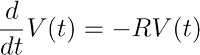(1)

The calculation of the intensities from the relaxation matrix requires to take the exponential of the matrix: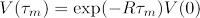(2)

To avoid heavy computations in the python scripts, ARIA relies on a different approach, which was proposed in Linge et al, 2004. For small time increments Δt, we can approximate the differential Eq. (1) for the NOE matrix V by a difference equation: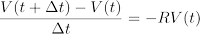(3)

Using the Eq. (3), it is possible to calculate the intensity matrix from the approximated Equation: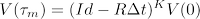(4)

where K is determined suwh that: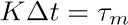The mean distance between two spins i and j is calculated from the distances measured on each structure of the ensemble: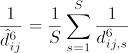(5)

where S denotes the number of structures in the ensemble. At the 0th iteration, the arithmetic ensemble average is used in place of Eq. (5) to avoid too strong weighting to the shortest distance.

### Calculation of the intensities

The integration of the relaxation matrix calculation into the ARIA procedure is straightforward: Structures of an iteration serve as templates for the calculation of NOE intensities. These are then used as correction factors in the determination of target distances for the next iteration. The necessary steps are:

1. Calculate matrix of all average inter-proton distances dij using Eq. (5)
2. Apply a distance cut-off criterion iteratively in order to obtain a sparse relaxatiion matrix. The cut-off criterion is that a relaxation el-
ement between two spins i and j is non-zero and included in the calculation if an intervening atom k is close to both of them:
3. Calculate relaxation matrix R
4. Calculate the NOEs using the iterative procedure of Eq. (4).

### Calibration of distance restraints

An approximate correction factor for every distance is then derived by applying a two-step calibration:

1. First a standard calibration to the experimental NOEs, using all observed peaks, to obtain Vkcalib: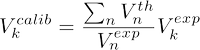(6)

where n runs over all experimental NOEs, which may be ambiguous. In that case, Vkth is the sum of all Vijth contributing to the ambiguous NOE k.

2. We then use the ratio of a calculated volume and its ISPA approximation to correct the target distance: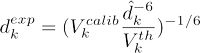(7)

where dk6 is the sum of all dij6 contributing to NOE k. By default, ARIA uses the calculated NOE intensities also to determine the contribution of each assignment possibility.

### Contribution cutoff

By default, ARIA uses the calculated NOE intensities also to determine the contribution of each assignment possibility. We define the contribution of each possible assignment to be proportional to the NOE intensity: Ca ∝ Vath where we normalize the contributions Ca such that: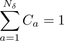where Nδ is the total number of contributions for a given frequency tolerance δ and the contributions are ordered according to size such that Ca ≥ Cb if a < b. We then partially assign NOEs by using these two equstions and the additional criterion: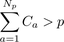in which p is the assignment cut-off and Np is the number of contributions to the peak necessary to exceed a given value of p. In other words, we remove the smallest contributions depending on the choice of the cut-off parameter p which is adjusted in each iteration. By default, p decreases from 1.0 in the first iteration to 0.8 in the last iteration.

The parameters p and Np are described by the attributes weight_threshold and max_contributions in the element partial_assignment of the project xml file. An example of the variation of these elements along a run is:

 Iteration # 0 1 2 3 4 5 6 7 8 p 1 0.9999 0.999 0.99 0.98 0.96 0.93 0.9 0.8

### Calculation of the error estimation

ARIA usually estimates the error of each distance from the size of the distance. Since most of the target distances will be corrected to larger values by the spin diffusion correction, this will also increase the error estimate. In order to take advantage of the improved
quality of the target distances, we introduce the option to base the error estimate on the calibrated experimental volume: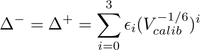(8)

with ε2 = 0.125 and ε0 = ε1 = ε3 = 0 by default. The net effect of using volume-based error bounds is to shift the whole interval [L, U] to larger volumes but not to increase the interval itself.

If the error on the distance is estimated from the size of the distance, the corresponding input in the project xml file is:

`<calibration relaxation_matrix="yes" distance_cutoff="6.0" estimator="ratio_of_averages"     error_estimator="distance"/>`

If the error is estimated from the volume, according to Eq. (8), the input is:

`<calibration relaxation_matrix="yes" distance_cutoff="6.0" estimator="ratio_of_averages"     error_estimator="volume"/>`

## Examples

Two examples of the use of the spin diffusion correction of distance are given on the Pleckstrin homology (PH) domain of Mus musculus β-spectrin (PDB structure). This protein corresponds to the example presented in the Linge et al, J Magn Reson 2004. The difference between both examples is the use of distance or of volume for the error estimation: the corresponding names for the archives are relax_dist.tgz and relax_vol.tgz, and the corresponding names for the project xml files are: ph_relax_dist.xml and ph_relax_volume.xml. The example names are: relax_dist and relax_vol.

The project directory tree is the same than those already described in the corresponding page.

## Input file format

The specificities of the input file required by the use of the spin diffusion correction, are all contained in the project xml file, and described below:

In each section iteration, the element calibration:

`<calibration relaxation_matrix="yes" distance_cutoff="6.0" estimator="ratio_of_averages"     error_estimator="volume"/>`

contains the parameters for the calibration with spin diffusion correction. The attribute relaxation_matrix describes whether the correction is enabled or not. The attribute distance_cutoff is used to filter the calculated intensities/volumes used for the calibration by including only those corresponding to a distance smaller than the distance_cutoff value. The attribute estimator defines how the calibration of experimental intensity with respect to the calculated ones is performed. The defalut value "ratio_of_averages" corresponds to a calibration through the ratio of the average experimental and calculated intensities/volumes, and is the only possible value for the moment. The attribte error_estimate tells whether the error is estimated on the target distance ("distance") or on the volume ("volume").

In the section spectrum, the element experiment_data:

`<experiment_data molecule_correlation_time="8.5" spectrum_mixing_time="80.0"   spectrometer_frequency="600.0"/>`

contains the molecule correlation time in ns, the spectrum mixing time in ms and the spectrometer frequecy in MHz. A molecule correlation time is defined for each spectrum, in order to take into account differences in experimental conditions and in samples.

« December 2023 »
December
MonTueWedThuFriSatSun
123
45678910
11121314151617
18192021222324
25262728293031